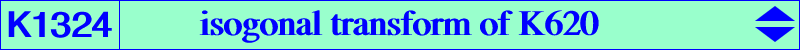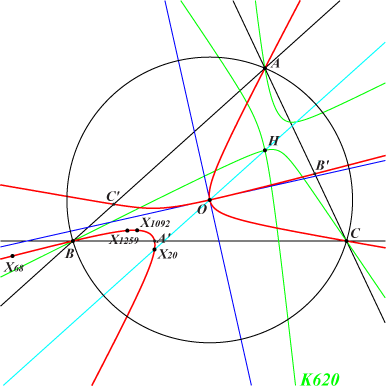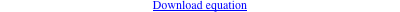too complicated to be written here. Click on the link to download a text file.X(3), X(X20), X(X68), X(X1092), X(X1259), X(X3926), X(X10316), X(X51254), X(53782), X(53783), X(53784), X(53785), X(53786), X(53787), X(53788), X(53789) isogonal conjugates of X(41320), X(43742) A', B', C' : vertices of the cevian triangle of X(69) Geometric properties :K1324 = psK(X4176, X69, X3) is the isogonal transform of K620 = psK(X6524, X2052, X4). K1324 is a nodal cubic with node O and nodal tangents parallel to the asymptotes of the Jerabek hyperbola. K1324 is the barycentric products X(394) × K028, X(69) × K260, X(3) × K935, X(3926) × K969. K1324 is also the Ω-isoconjugate of the cubic K for these {Ω, K} : {X3, K555}, {X6, K620}, {X184, K429}, {X394, K257}, {X577, K009}, {X50433, K724}. K1324 meets the circum-circle at the same points as pK(X6, X11413) where X(11413) is the reflection of X(24) in X(3). K1324 meets the line at infinity at the same points as pK(X6, P) where P is the intersection of the lines {X2, X578}, {X4, X52}, {X5, X1993}, {X20, X1204}, {X70, X265}, {X155, X403}, etc, SEARCH = -1.56602832643679.